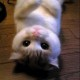# programming in lua (2)

3-4 章。

lua 支持的数值运算符有：“+”（加），“-”（减），“*”（乘），“/”（除），“^” （指数），“%”（求余），“-”（取相反数）。“%”还有取特定精度的作用，例如“x%1”表示取 x 的整数部分，而“x%0.01”则取到小数点后两位。

``````^
not # -（取相反数）
* / %
+ -
..
< > <= >= ~= ==
and
or
``````

table 的初始化使用“{}”或者在“{}”中包含初始值：

``````letters = {"a", "b", "c", "d", "e"}
print(letters) -- "c"
``````

table 下标从 1 开始（看来如果不显式指定 key 的值默认用下标代替 key）。另外也可以使用下面的语法进行初始化：

``````a = {x = 10, y = 20}
b = {}; b.x = 10; b.y = 20
print(a["x"]) -- 10
``````

a 和 b 的初始化方法是等价的。初始化还可以嵌套：

``````a = {
{x = 10, y = 20},
{x = -10, y = -20}
}
print(a["y"]) -- -20
``````

``````opnames = {["+"] = "add", ["-"] = "sub"}
a = {[-2] = 100, [-1] = 10}
print(opnames["+"]) -- "add"
print(a[-1]) -- 10
``````

``````a = { = "a", "b", "c", "d", "e"}
print(a) -- a
print(a) -- b
``````

“b”的下标从 1 开始，因为它是列表的第一个元素（因为“a”是指定了 key 为 0 的元素，而除了“a”之外都没指定 key，默认就从 1 开始编号了）。

lua 中的函数中默认数组下标从 1 开始，所以最好不要指定下标从 0 开始。

``````a = {x = 10; y = 45; "one", "two", "three",}
print(a["x"]) -- 10
print(a) -- "two"
``````

``````x, y = 10, 20
print(x) -- 10
print(y) -- 20
``````

lua 会先将等号右边的值都计算出来，再进行赋值操作，所以交换两个元素的值可以这样写：

``````x,y = y, x
``````

``````x, y, z = 10, 20 -- x = 10, y = 20, z = nil
x, y = 10, 30, 50 -- x = 10, y = 30
``````

``````if condition then
do something
end
``````

``````if condition1 then
do something
elseif condition2 then
do something
end
``````

while 循环的语法：

``````while condition do
do something
end
``````

repeat 循环的语法：

``````repeat
do something
until condition
``````

for 循环有两种，一种是数值型的，语法是

``````for var = exp1, exp2, exp3 do
do something
end
``````

``````for i = 1, 10, 2 do
print(i)
end
``````

``````days = {"Sun", "Mon", "Tue", "Wed", "Thu", "Fri", "Sat"}

for k, v in pairs(days) do
print(v, k)
end
``````

``````for var = 1, 10, 2 do
local i
i = var + 1
print(i)
end

print(i) -- nil
``````

``````a = {"a", "b", "c", "d", "e"}

i = 1
while a[i] do
if a[i] == "c" then
break
end
i = i + 1
end

print("i =", i)
``````

return 可以立即终止当前函数。每个函数的最后都隐含有一个 return 语句。

## Comments (2)

1.lcc说道：

小子学习很用功啊

1.ou说道：

不用功进不了你的公司啊